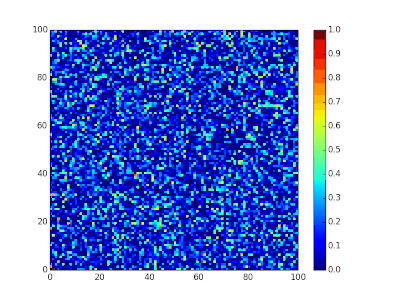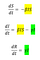# Modeling an Epidemic

## A Mathematical Perspective

hile I am devastated about the tragic deaths around the world due to COVID-19, I wanted to know how we can predict/ model an epidemic in scientific computing. Turns out It is not that big of a problem if you know some basic laws and initial conditions, well… that’s pretty much true for any problem I guess.

There are many ways to do this, obviously, to keep this article simple and beginner friendly, we will look into most popular of them all, the SIR model.

# SIR Model

Before understanding how this model works, let’s get familiar with the terms in use.

• S — number of susceptible (likely or liable to be influenced or harmed by the disease)
• I — number of infectious (liable to spread infection)
• R — number of recovered or deceased (or immune) individuals

Susceptible is a group of people who are vulnerable to exposure with infectious people. They can be patient when the infection happens. The group of infectious represents the infected people. They can pass the disease to susceptible people and can be recovered in a specific period. Recovered people get immunity so that they are not susceptible to the same illness anymore. SIR model is a framework describing how the number of people in each group can change over time.

We can model this scenario with system of differential equations.

β is a parameter controlling how much the disease can be transmitted through exposure. It is determined by the chance of contact and the probability of disease transmission. γ is a parameter expressing how much the disease can be recovered in a specific period. Once the people are healed, they get immunity. There is no chance for them to go back susceptible again.

We are making few assumption before we get into modeling, the first assumption that we make is that the epidemic is sufficiently short so it doesn’t last that long so that we can assume that the total population remains constant. (We do not consider the effect of the natural death or birth rate because the model assumes the outstanding period of the disease is much shorter than the lifetime of the human.)

Second assumption in our model relates to the way in which the disease is transmitted and we assume that the rate of increase in the infectives is proportional to the contact between susceptibles and infectives and we assume that this occurs at a constant rate.

Third assumption relates to the removal rate and we’re going to assume that there is a constant rate. This could be a death rate or a recovery rate.Spatial SIR model simulation. Each cell can infect its eight immediate neighbors. Source — Wikipedia3 Differential equations that governs SIR model.

In the first equation the sign is (-) for βIS because number of susceptible individuals decline overtime. Those who get infected because of this are moved to the Infected group (βIS). From that group number of Recovered (or Removed) individual will be added to Recovered group (γI).

When we can estimate the two values (β and γ), there are several insights derived from it. If the D is the average days to recover from infectious, it is derived from γ.

Also, we can estimate the nature of the disease in terms of the power of infection. It is called a basic reproduction number. Rₒ is the average number of people infected from one other person. If it is high, the probability of pandemic is also higher.

In an epidemic (now pandemic) like COVID-19 the best approach is SEIR because it has the “E — Exposed” group who are pre-infectious. They do not show symptoms but actively contribute to spread the disease. But as I mentioned earlier, to keep the model simple we will stick with SIR. Although we will make a small modification to show you how we can adapt and change this model to different scenarios.

As mentioned earlier, the ‘R’ in SIR are the number of recovered or deceased (or immune) individuals. We will split this to 2 groups, one for recovered (R) and one for deceased (or dead), some of the infected will recover while others will die.

We will call the rate of individuals transitioning from infected to deceased (D) as α.

Now you can start with a population, define α, β and γ, and see how it is going to behave.

# Modeling

There is not enough epidemiological information at this time to determine how easily this virus spreads between people, but it is currently estimated that, on average, reproductive number of COVID-19 is between 1.4–3.9 [source]. We will do this for Sri Lanka, by April 6th 2020, the country recognized Total Number Confirmed — 176, Total Number Recovered — 34, Total Number of Deaths — 5. [source]. I’m a technologist, not an epidemiologist. So we will carry on based on these numbers, these can change in the future and sole purpose of this article is to show you how we model problems like this using algorithms with the help of mathematics, not about facts.

Based on above numbers, we will assume,

α = (Local Deaths / Local Total Cases) = 5/176 = 0.028

γ = (Local Recovered / Local Total Cases) = 34/176 = 0.193

β = basic reproduction number * γ

I will implement this in python, all you need to do is define an array of time steps, if you want to model this for 40 days you should have a 2D array, with 40 columns and 4 rows (because 4 equations; to hold solution for all 4 equations at each time step), and then solve the equations for each time step, 40 times. (code should be self explanatory, I have added comments to everywhere possible, go through it)

I have limited the population for 100 people, for basic reproduction number 1.4, this is the output.

You can see that within 1 day, all 100 susceptible humans became infected and total number of deaths went up to nearly 13. This is assuming the healthcare system can handle all 100 people. But in real world this is not the case. When the social distancing protocols in place, we can reduce the basic reproduction number, so it helps to flatten the curve. As an example, if I were to reduce basic reproduction number to 0.05,

maximum number of infected humans reduced to 45, while in previous case all 100 were infected.

So that’s it. Hope you learned something new. 3Blue1Brown has an amazing visual simulations if you want to deep dive, do check it out.

If you want to learn more about SIR model, and some other ways to implement the model, go through following resources.

Systems Design • Social Innovation • Cloud • ML

## More from Nishan Chathuranga Wickramarathna

Systems Design • Social Innovation • Cloud • ML